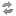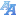Exam dates(version: 176)
Browse before 2018/2019 summer
Login via CASType: all courses ---Environment CentreCentre for Theoretical Study;Institute of the History of Charles University and Archive of Charles UniversityRectorateCatholic Theological FacultyProtestant Theological FacultyHussite Theological FacultyFaculty of LawFirst Faculty of MedicineSecond Faculty of MedicineThird Faculty of MedicineFaculty of Medicine in PilsenFaculty of Medicine in Hradec KrálovéFaculty of Pharmacy in Hradec KrálovéFaculty of ArtsFaculty of ScienceFaculty of Mathematics and PhysicsFaculty of EducationFaculty of Social SciencesFaculty of Physical Education and SportFaculty of HumanitiesInstitute for Language and Preparatory StudiesCentral Library of Charles UniversityCentre for Economic Research and Graduate EducationCentre for Knowledge and Technology Transfer---Astronomical Institute of Charles University (32-AUUK)Blíže nespecifikované praxe (32-#PRX)Computer Science Institute of Charles University (32-IUUK)Department of Algebra (32-KA)Department of Applied Mathematics (32-KAM)Department of Atmospheric Physics (32-KFA)Department of Condensed Matter Physics (32-KFKL)Department of Distributed and Dependable Systems (32-KDSS)Department of Geophysics (32-KG)Department of Chemical Physics and Optics (32-KCHFO)Department of Language Education (32-KJP)Department of Low Temperature Physics (32-KFNT)Department of Macromolecular Physics (32-KMF)Department of Mathematical Analysis (32-KMA)Department of Mathematics Education (32-KDM)Department of Numerical Mathematics (32-KNM)Department of Physical Education (32-KTV)Department of Physics Education (32-KDF)Department of Physics of Materials (32-KFM)Department of Probability and Mathematical Statistics (32-KPMS)Department of Software and Computer Science Education (32-KSVI)Department of Software Engineering (32-KSI)Department of Surface and Plasma Science (32-KFPP)Department of Theoretical Computer Science and Mathematical Logic (32-KTIML)Institute of Formal and Applied Linguistics (32-UFAL)Institute of Particle and Nuclear Physics (32-UCJF)Institute of Physics of Charles University (32-FUUK)Institute of Theoretical Physics (32-UTF)Laboratory of General Physics Education (32-KVOF)Matematický ústav AV ČR, v.v.i. (32-MUAV)Mathematical Institute of Charles University (32-MUUK)Network and Labs Management Center (32-SISAL)Student Affairs Department (32-STUD)Ústav teorie informace a automatizace AV ČR, v.v.i. (32-UTIAAV)---Selected Topics on Mathematics for Physicists - NMAF006Advanced Linear Algebra for Physicists - NMAF037Proseminar on Mathematical Analysis - NMAI068Open Problem Seminar - NMAT057Seminar for bachelor thesis - NMAT362Mathematical Analysis 1 - NMMA101Mathematical Analysis 2 - NMMA102Calculus 1 - NMMA111Calculus 2 - NMMA112Proseminar on Mathematical Analysis - NMMA161Proseminar on Mathematical Analysis - NMMA162Metric Spaces - NMMA164Mathematical Analysis 3 - NMMA201Mathematical Analysis 4 - NMMA202Measure and Integration Theory - NMMA203Calculus 3 - NMMA211Calculus 4 - NMMA212Proseminar on Mathematical Analysis 3 - NMMA261Proseminar on Mathematical Analysis 4 - NMMA263Introduction to Complex Analysis - NMMA301Introduction to Functional Analysis - NMMA331Ordinary Differential Equations - NMMA333Introduction to Partial Differential Equations - NMMA334General Topology 1 - NMMA335Seminar on Real Functions Theory 1 - NMMA337Complex Analysis 1 - NMMA338Seminar on Real Functions Theory 2 - NMMA340Selected Topics on Functional Analysis - NMMA342Metric Structures - NMMA361Topology of a continuum - NMMA363Seminar on Basic Properties of Function Spaces - NMMA365Mathematical methods in Practice - NMMA366Functional Analysis 1 - NMMA401Functional Analysis 2 - NMMA402Theory of Real Functions 1 - NMMA403Theory of Real Functions 2 - NMMA404Ordinary Differential Equations 2 - NMMA407Complex Analysis 2 - NMMA408Seminar on Differential Equations - NMMA431Topological Methods in Functional Analysis 1 - NMMA435Topological Methods in Functional Analysis 2 - NMMA436Seminar on Function Spaces - NMMA454Seminar on Real and Abstract Analysis - NMMA455Seminar on Real Functions Theory - NMMA456Seminar on Topology - NMMA458Seminar on Fundamentals of Functional Analysis - NMMA459General Topology 2 - NMMA462Problem solving seminar - NMMA465Applications of Differential Equations in Biology - NMMA466An introduction to mathematical homogenization - NMMA469Chapters from discrete dynamical systems - NMMA479Mathematical Analysis Elective 1 - NMMA498Nonlinear Functional Analysis 1 - NMMA501Nonlinear Functional Analysis 2 - NMMA502Introduction to Interpolation Theory 1 - NMMA533Introduction to Interpolation Theory 2 - NMMA534Advanced Differentiation and Integration 3 - NMMA563Advanced Differentiation and Integration 4 - NMMA564Topics in Dynamical Systems - NMMA574Topological and Geometric Properties of Convex Sets 1 - NMMA575Topological and Geometric Properties of Convex Sets 2 - NMMA576Mathematics 1 - NMMA701Mathematics 2 - NMMA702Mathematics 3 - NMMA703Mathematics 4 - NMMA704Mathematics 5 - NMMA705Applied differential equations - NMMA706Mathematics 1 - NMMA711Mathematics 2 - NMMA712Introductory Mathematics - NMMA713Introduction to Complex Analysis (O) - NMMA901Measure and Integration Theory (O) - NMMA903Introduction to Functional Analysis (O) - NMMA931Selected Topics on Functional Analysis (O) - NMMA942---Bárta Tomáš, doc. RNDr., Ph.D.Benešová Barbora, Mgr., Ph.D.Cúth Marek, Mgr., Ph.D.Hencl Stanislav, prof. RNDr., Ph.D.Holický Petr, doc. RNDr., CSc.Honzík Petr, Mgr., Ph.D.Johanis Michal, doc. RNDr., Ph.D.Kalenda Ondřej, prof. RNDr., Ph.D., DSc.Malý Jan, prof. RNDr., DrSc.Opic Bohumír, doc. RNDr., DrSc.Pick Luboš, prof. RNDr., CSc., DSc.Pražák Dalibor, doc. RNDr., Ph.D.Pyrih Pavel, doc. RNDr., CSc.Rokyta Mirko, doc. RNDr., CSc.Spurný Jiří, prof. RNDr., Ph.D., DSc.Vlasák Václav, RNDr., Ph.D.Zajíček Luděk, prof. RNDr., DrSc.Zelený Miroslav, doc. RNDr., Ph.D. only future only the free ones 20501002505001000
* no data found

Charles University | Information system of Charles University | http://www.cuni.cz/UKEN-329.html